• 一、简介 VRP问题描述： 假设在一个供求关系系统中，车辆从货源取货，配送到对应的若干配送点。车辆存在最大载货量，且配送可能有时间限制。需要合理安排取货时间，组织适当的行车路线，使用户...C-W节约算法： 基本

一、VRP简介

1 VRP基本原理
车辆路径规划问题(Vehicle Routing Problem，VRP)是运筹学里重要的研究问题之一。VRP关注有一个供货商与K个销售点的路径规划的情况，可以简述为：对一系列发货点和收货点，组织调用一定的车辆，安排适当的行车路线，使车辆有序地通过它们，在满足指定的约束条件下（例如：货物的需求量与发货量，交发货时间，车辆容量限制，行驶里程限制，行驶时间限制等），力争实现一定的目标（如车辆空驶总里程最短，运输总费用最低，车辆按一定时间到达，使用的车辆数最小等）。
VRP的图例如下所示：2 问题属性与常见问题
车辆路径问题的特性比较复杂，总的来说包含四个方面的属性：
（1）地址特性包括：车场数目、需求类型、作业要求。
（2）车辆特性包括：车辆数量、载重量约束、可运载品种约束、运行路线约束、工作时间约束。
（3）问题的其他特性。
（4）目标函数可能是总成本极小化，或者极小化最大作业成本，或者最大化准时作业。

3 常见问题有以下几类：
（1）旅行商问题
（2）带容量约束的车辆路线问题(CVRP)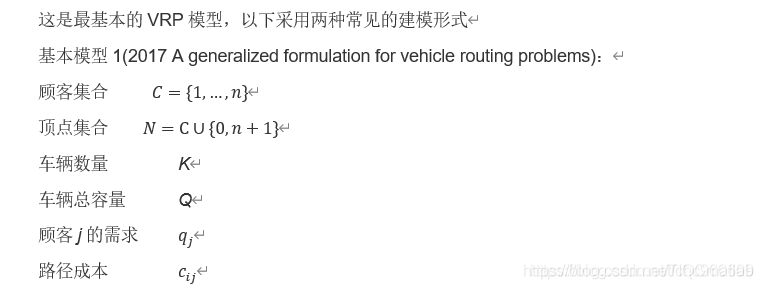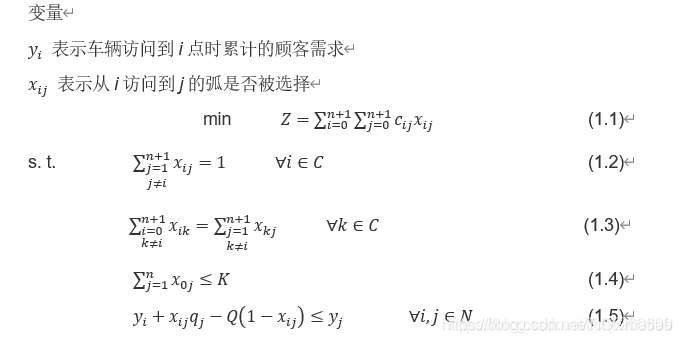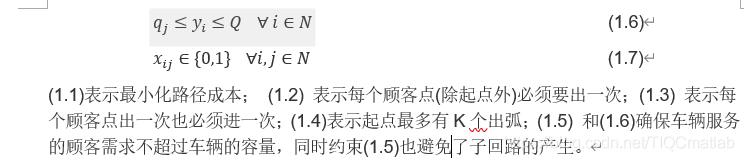该模型很难拓展到VRP的其他场景,并且不知道具体车辆的执行路径，因此对其模型继续改进。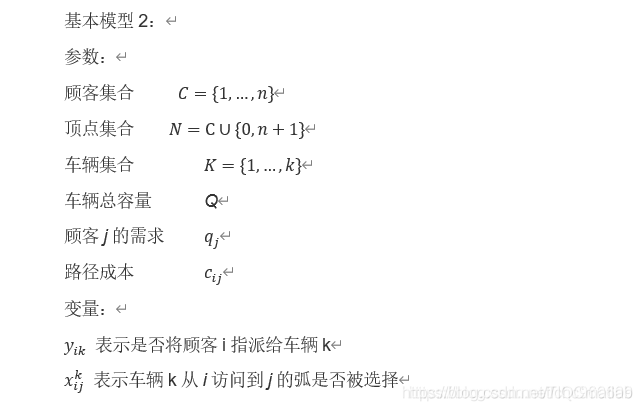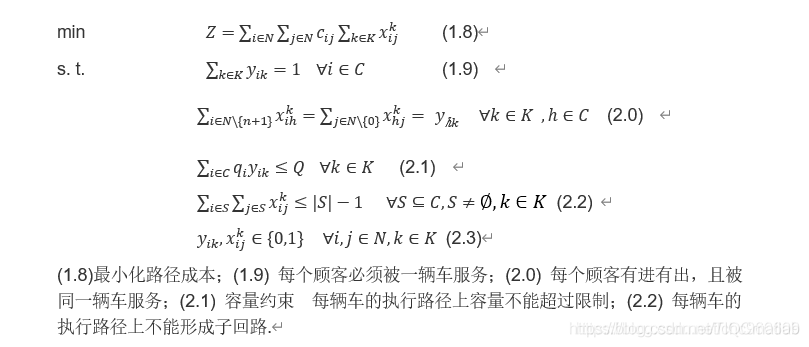（3）带时间窗的车辆路线问题
由于VRP问题的持续发展，考虑需求点对于车辆到达的时间有所要求之下，在车辆途程问题之中加入时窗的限制，便成为带时间窗车辆路径问题（VRP with Time Windows, VRPTW）。带时间窗车辆路径问题（VRPTW）是在VRP上加上了客户的被访问的时间窗约束。在VRPTW问题中，除了行驶成本之外, 成本函数还要包括由于早到某个客户而引起的等待时间和客户需要的服务时间。在VRPTW中，车辆除了要满足VRP问题的限制之外，还必须要满足需求点的时窗限制，而需求点的时窗限制可以分为两种，一种是硬时窗（Hard Time Window），硬时窗要求车辆必须要在时窗内到达，早到必须等待，而迟到则拒收；另一种是软时窗（Soft Time Window），不一定要在时窗内到达，但是在时窗之外到达必须要处罚，以处罚替代等待与拒收是软时窗与硬时窗最大的不同。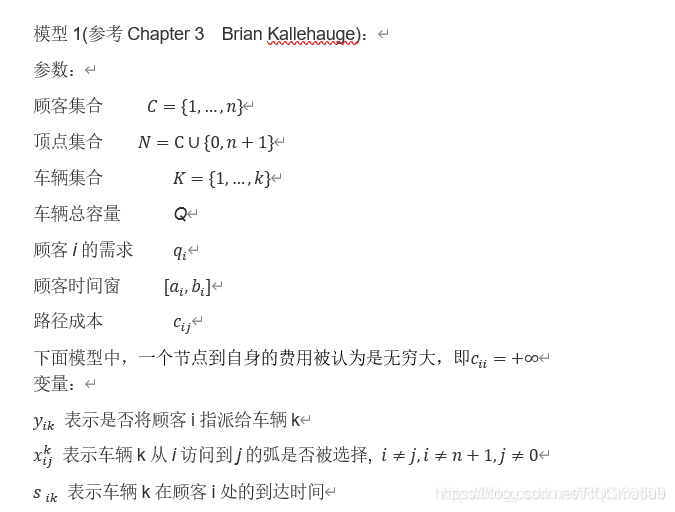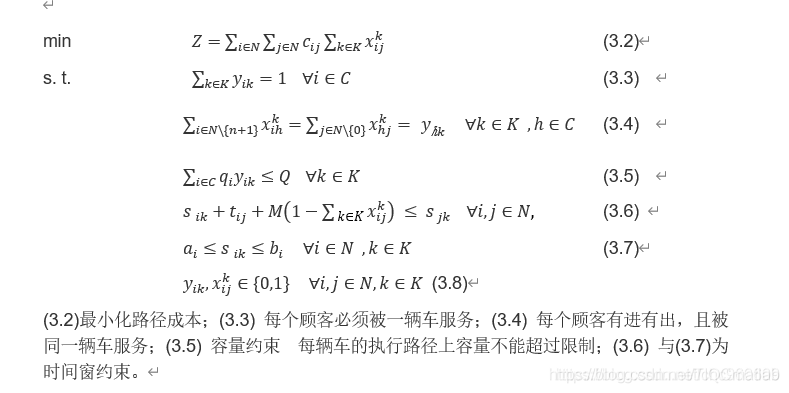模型2(参考2017 A generalized formulation for vehicle routing problems)：
该模型为2维决策变量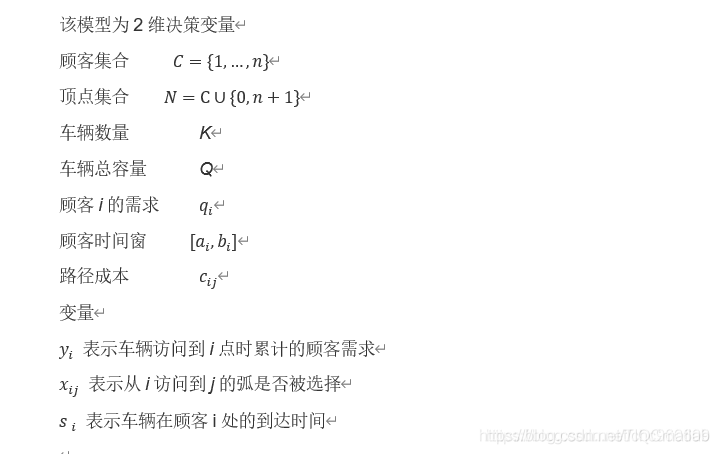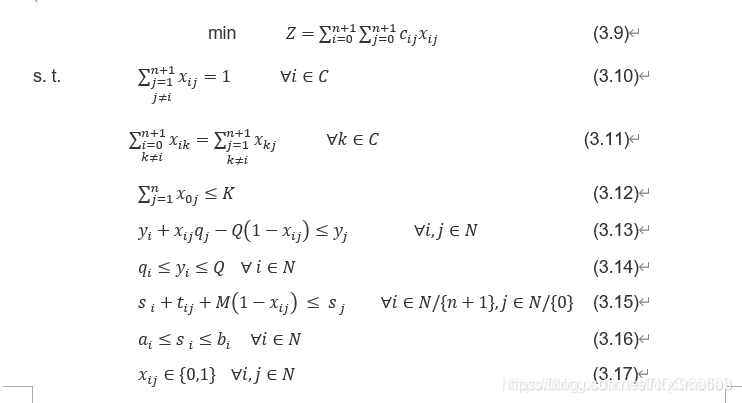（4）收集和分发问题
（5）多车场车辆路线问题
参考(2005 lim，多车场车辆路径问题的遗传算法_邹彤, 1996 renaud)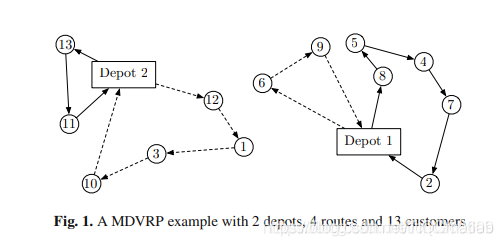由于车辆是同质的，这里的建模在变量中没有加入车辆的维度。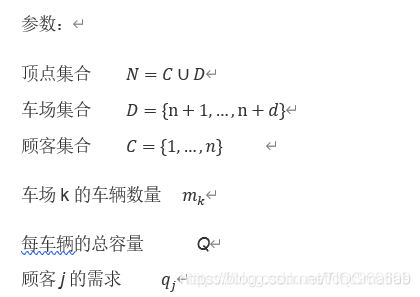（6）优先约束车辆路线问题
（7）相容性约束车辆路线问题
（8）随机需求车辆路线问题

4 解决方案
（1）数学解析法
（2）人机交互法
（3）先分组再排路线法
（4）先排路线再分组法
（5）节省或插入法
（6）改善或交换法
（7）数学规划近似法
（8）启发式算法

5 VRP与VRPTW对比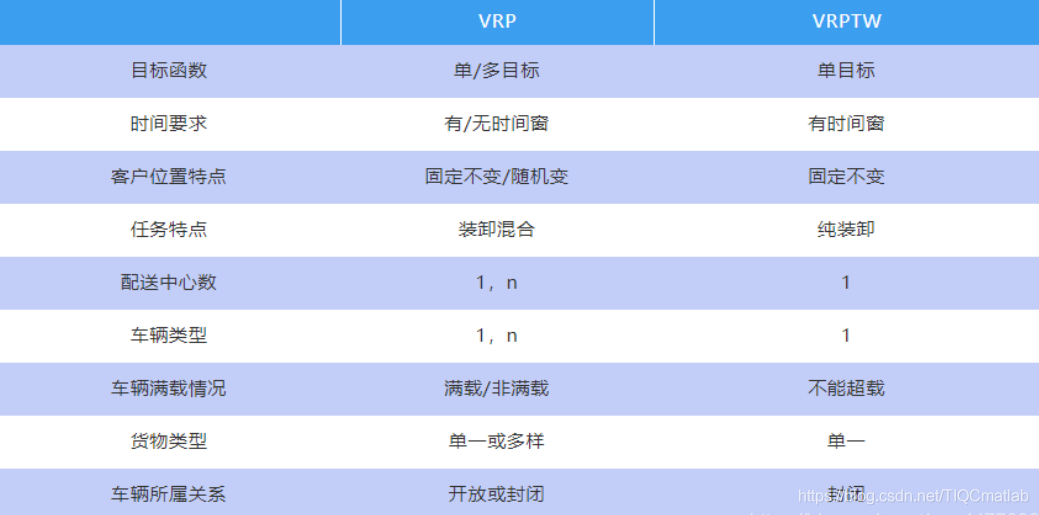二、节约算法简介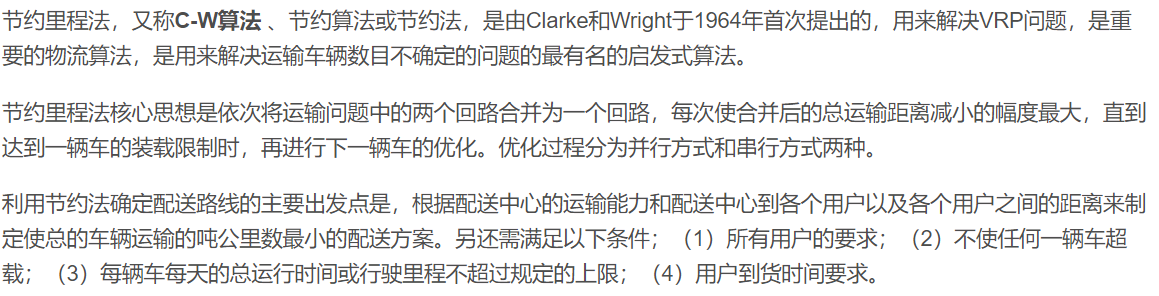基本思想行时通过这一条弧。

迭代步骤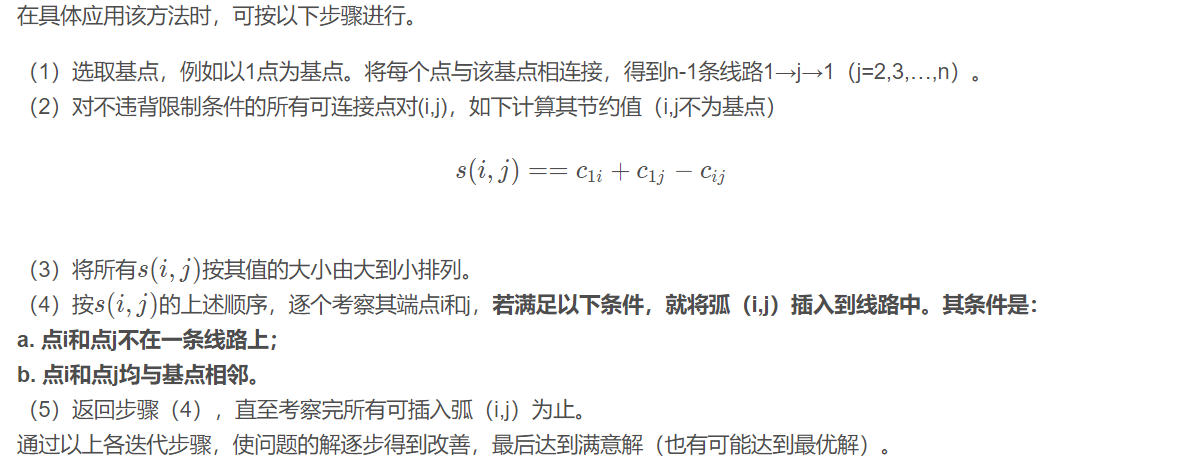三、部分源代码

clear
clc
tic
%% 用importdata这个函数来读取文件
rc208=importdata('rc208.txt');
cap=1000;
%% 提取数据信息
vertexs=rc208(:,2:3);                                            %所有点的坐标x和y
customer=vertexs(2:end,:);                                       %顾客坐标
cusnum=size(customer,1);                                         %顾客数
demands=rc208(2:end,4);                                          %需求量
h=pdist(vertexs);
dist=squareform(h);                                             %距离矩阵，满足三角关系，暂用距离表示花费c[i][j]=dist[i][j]
%% CW法构造CVRP初始解
[init_vc,init_TD,init_vl]=init_CVRP(rc208,cap);
initNV=size(init_vc,1);
str1=['车辆行驶总距离 =  ' num2str(init_TD)];
disp(str1)
str2=['车辆使用数目 =  ' num2str(initNV)];
disp(str2)
%% 判断最优解是否满足时间窗约束和载重量约束，0表示违反约束，1表示满足全部约束
flag=Judge(init_vc,cap,demands);
%% 检查最优解中是否存在元素丢失的情况，丢失元素，如果没有则为空
DEL=Judge_Del(init_vc);
%% 画出配送路线图
vertexs=rc208(:,2:3);                                            %所有点的坐标x和y
draw_Best(init_vc,vertexs);
toc

四、运行结果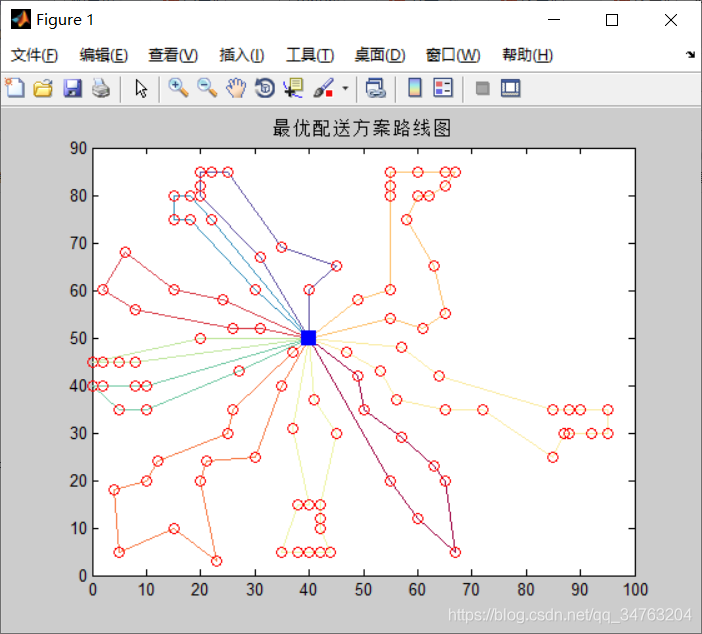五、matlab版本及参考文献

1 matlab版本
2014a

2 参考文献
 包子阳,余继周,杨杉.智能优化算法及其MATLAB实例（第2版）[M].电子工业出版社，2016.
张岩,吴水根.MATLAB优化算法源代码[M].清华大学出版社，2017.

展开全文• 一、节约算法简介 基本思想 行时通过这一条弧。 迭代步骤 二、部分源代码 clc clear all p1=0.9; customer=xlsread('customer.xlsx'); %需求点信息 facility=xlsread('facility.xlsx'); %设施点信息 ...

一、节约算法简介基本思想行时通过这一条弧。

迭代步骤二、部分源代码

clc
clear all
p1=0.9;
facilityposition=facility(:,2:3);               %设施坐标
customerposition=customer(:,2:3);               %需求点坐标
position=[facilityposition;customerposition];
xlswrite('position.xlsx',position)
position1=[position(:,1) position(:,2)];
distMatrix=dists(position1);                    %计算得出的两点之间的距离
xlswrite('distMatrix.xlsx',distMatrix)

ttimeu=fix(distMatrix);                         %两点之间的距离
%%%%%%%%%%%%%%%%%%%%%%%%%固定数据%%%%%%%%%%%%%%%%%%%%%%%%%%%%%%%%%%%%
Qofcar=200;                                     %车辆容量
costofallcar=5000;                               %车辆固定成本
costofunitdistance=9;                            %单位距离成本
tanpaifangyinzi=1;                               %车辆碳排放因子
danweiyouhao=1;                                  %车辆单位油耗
%%%%%%%%%%%%%%%%%%%%%%%计算出来的数据%%%%%%%%%%%%%%%%%%%%%%%%%%%%%%%%
Numberofpoints=size(customer,1);                %需求点数量
Numberoffacilities=size(facility,1);            %设施点数量
quantity=[customer(:,1) customer(:,4) customer(:,4)];     %需求点需求量
Qoffacilities=[facility(:,1) facility(:,4)];    %设施容量
timewindow=[customer(:,1) customer(:,6:7)];     %需求点时间窗
countfacility=facility(:,5);                    %建立设施固定成本
codeofpicture=1;

timewindow

assignofpoint=[2 1 2 1 3 3 1 2 1 1 2 3 2];
[outcome1,outcome2,outcome3]=cw(Numberoffacilities,assignofpoint,ttimeu,timewindow,distMatrix,quantity,Qofcar,p1);
%outcome1=[1 1 2 1 2 3 3 4 4 3 5 5 5];
%outcome2=[9 2 10 4 7 13 1 11 8 3 12 5 6];
[outcome1,outcome2,outcome3]=tabu(outcome1,outcome2,outcome3,distMatrix,ttimeu,Numberoffacilities,timewindow);

[Picture]=picture(codeofpicture,outcome1,outcome2,outcome3,customer,facility);
function [outcome1,outcome2,outcome3]=cw(Numberoffacilities,assignofpoint,ttimeu,timewindow,distMatrix,quantity,Qofcar,p1)
H=1;
outcome3=zeros(1,Numberoffacilities);%与设施点的伪编号是一一对应的
%%%%%%%找到实际分配的设施点，因为之前的chrom中为1的点，可能%%%%%%%%%%%%%
facilitypop=zeros(1:Numberoffacilities);
facilitypop(assignofpoint)=1;
trueSelectefacilities=find(facilitypop(1,:)==1);     %被选择的设施编号
trueselectNumberoffacilities=size(trueSelectefacilities,2);
outcome1=cell(1,trueselectNumberoffacilities);
outcome2=cell(1,trueselectNumberoffacilities);
for i=1:trueselectNumberoffacilities%此时的设施为i
a=trueSelectefacilities(i);%设施的伪编号
pointofsubroute=find(assignofpoint==a);%属于该设施点的所有需求点的伪编号
Numberofpointsofsubroute=size(pointofsubroute,2);%该设施点的需求点数量
judge=zeros(1,Numberofpointsofsubroute);                           %判断需求点的位置情况
chrom1=zeros(1,Numberofpointsofsubroute);%最后输入的是伪编号
chrom2=sort(pointofsubroute);                                      %需求点伪编号从小到大排序
originalchrom2=chrom2;

%%对关键节点先分配一个车辆给它？？？？？？？？？？？？？？？？？？？？？对不对？
%for j=1:Numberofpointsofsubroute
%if timewindow(chrom2(j),2)==timewindow(chrom2(j),3)
%chrom1(j)=H;
%H=H+1;
%end
%end
%%%%%%%%计算到达时间%%%%%%%%%%%%%%%%%%%%
arrivetime=zeros(1,Numberofpointsofsubroute);%需求点的到达时间,与当前的originalchrom2位置一一对应，与分配给该设施点的需求点一一对应
for j=1:Numberofpointsofsubroute
if ttimeu(chrom2(j)+Numberoffacilities,a)<timewindow(chrom2(j),2)
arrivetime(j)=timewindow(chrom2(j),2);
else
arrivetime(j)=ttimeu(chrom2(j)+Numberoffacilities,a);
end
end
%%%%%%%%%%%%%%%%%%%%%%%%%路径节约值列表%%%%%%%%%%%%%%%%%%%%%%
savingnumber=zeros(Numberofpointsofsubroute);%与分配给该设施的需求点的伪编号一一对应
for j=1:Numberofpointsofsubroute
for z=1:Numberofpointsofsubroute
if j~=z
savingnumber(j,z)=distMatrix(chrom2(j)+Numberoffacilities,a)+distMatrix(chrom2(z)+Numberoffacilities,a)-distMatrix(chrom2(j)+Numberoffacilities,chrom2(z)+Numberoffacilities);
end
end
end
[a b]=max(savingnumber);%a输入值，b中对应的数字为行，对应的列数为列
[c d]=max(a);%c输入具体的当前节约值，d为列数
e=b(d);%e为行
%%%在节约值列表中e行d列是当前最大的节约量
E=chrom2(e);%此时的E为节约量最大的需求点伪编号之一
D=chrom2(d);%此时的D为节约量最大的需求点伪编号之1
done=1;
while(done<2)
if judge(e)==0&&judge(d)==0
PD=0;
a=quantity(E,2)+quantity(D,2);
aa=quantity(E,3)+quantity(D,3);
pp1=normcdf(Qofcar,a,sqrt(aa));
a2=find(chrom2==D);
b2=find(chrom2==E);
if pp1>p1
EFj=arrivetime(e)+ttimeu(E+Numberoffacilities,D+Numberoffacilities)-arrivetime(d);
if EFj<0
PD=1;
end
elseif EFj==0
PD=1;
elseif EFj>0
delaytime=timewindow(D,3)-arrivetime(d);
if delaytime>=EFj
PD=1;
end
end
if PD==1
chrom1(e)=H;
chrom1(d)=H;%H为车辆编号
judge(e)=1;%与设施直接相连的起点为1
judge(d)=2;%与设施直接相连的终点为2
H=H+1;
arrivetime(d)=arrivetime(d)+EFj;
if b2>a2
chrom2(a2)=E;
chrom2(b2)=D;
end
end
end
savingnumber(e,d)=0;
elseif judge(e)==0&&judge(d)==1;
a2=find(chrom2==D);
b2=find(chrom2==E);
a=find(chrom1==chrom1(a2));
b=sum(quantity(chrom2(a),2))+quantity(E,2);
bb=sum(quantity(chrom2(a),3))+quantity(E,3);
pp1=normcdf(Qofcar,b,sqrt(bb));
c=size(a,2);
if pp1>p1
EFj=arrivetime(e)+ttimeu(E+Numberoffacilities,D+Numberoffacilities)-arrivetime(d);
if EFj<0
a1=10000;
for j=1:c
b1=find(originalchrom2==chrom2(a(j)));
end
end
PD=1;
end
elseif EFj==0
PD=1;
elseif EFj>0
a1=10000;
for j=1:c
b1=find(originalchrom2==chrom2(a(j)));
Delaytime=timewindow(chrom2(a(j)),3)-arrivetime(b1);
if Delaytime<a1
delaytime=Delaytime;
a1=Delaytime;
end
end

三、运行结果四、matlab版本及参考文献

1 matlab版本
2014a

2 参考文献
 包子阳,余继周,杨杉.智能优化算法及其MATLAB实例（第2版）[M].电子工业出版社，2016.
张岩,吴水根.MATLAB优化算法源代码[M].清华大学出版社，2017.

展开全文matlab 算法 开发语言
• 求助节约算法MATLAB代码

千次阅读 2020-12-19 14:18:42
end end %% 计算节约里程项目 for i =1: 1: length(points) for j =i: 1: length(points)-1 if j; end PA{i,j} = path{i,j}.distance; PB{i,j}= path{i,j+1}.distance; A=j-1; B=j; A_=A+1; B_=B+1 AB{i,j}= path{A...

该楼层疑似违规已被系统折叠 隐藏此楼查看此楼

%% 生成路径数据

data=[

[0,10,9,7,8,8,8,3,4,10,7],

[0,0,4,9,14,18,18,13,14,11,4],

[0,0,0,5,10,14,17,12,13,15,8],

[0,0,0,0,5,9,15,10,11,17,13],

[0,0,0,0,0,6,13,11,12,18,15],

[0,0,0,0,0,0,7,10,12,18,15],

[0,0,0,0,0,0,0,6,8,17,15],

[0,0,0,0,0,0,0,0,2,11,10],

[0,0,0,0,0,0,0,0,0,9,11],

[0,0,0,0,0,0,0,0,0,0,8],

[0,0,0,0,0,0,0,0,0,0,0]

];

P=Node();P.name='P';

points={P};

for i=1: 1:10

x=Node();

x.name=char('A'+i-1);

points{i+1}=x;

end

for i =1: 1: length(points)

for j =1: 1: length(points)

if j

continue;

end

x=Node();

x.name=[points{i}.name ,'-' , points{j}.name];

x.pointA=points{i};

x.pointB=points{j};

x.distance=data(i,j);

path{i,j}=x;

end

end

%% 计算节约里程项目

for i =1: 1: length(points)

for j =i: 1: length(points)-1

if j<=i

continue;

end

PA{i,j} = path{i,j}.distance;

PB{i,j}= path{i,j+1}.distance;

A=j-1;

B=j;

A_=A+1;

B_=B+1

AB{i,j}= path{A_,B_}.distance;

PAB{A-i+1,B}=PA{i,j}+PB{i,j}-AB{i,j};

end

asdf=9;

end

第二步 规则不清。遇到困难

展开全文• 202104节约里程法模拟退火遗传算法求解车辆路径问题Matlab视频教程配套资料.pdf
• 使用模拟退火算法对里程问题进行优化，并给出合理的解
• 一、简介 车辆路径问题（VRP） 车辆路径问题（VRP）最早是由 Dantzig 和 Ramser 于1959年首次提出，它是指一定数量的客户，各自有不同数量的货物需求，配送中心向客户提供货物，由一个车队负责分送货物，组织适当的...

一、VRP简介

1 VRP基本原理
车辆路径规划问题(Vehicle Routing Problem，VRP)是运筹学里重要的研究问题之一。VRP关注有一个供货商与K个销售点的路径规划的情况，可以简述为：对一系列发货点和收货点，组织调用一定的车辆，安排适当的行车路线，使车辆有序地通过它们，在满足指定的约束条件下（例如：货物的需求量与发货量，交发货时间，车辆容量限制，行驶里程限制，行驶时间限制等），力争实现一定的目标（如车辆空驶总里程最短，运输总费用最低，车辆按一定时间到达，使用的车辆数最小等）。
VRP的图例如下所示：2 问题属性与常见问题
车辆路径问题的特性比较复杂，总的来说包含四个方面的属性：
（1）地址特性包括：车场数目、需求类型、作业要求。
（2）车辆特性包括：车辆数量、载重量约束、可运载品种约束、运行路线约束、工作时间约束。
（3）问题的其他特性。
（4）目标函数可能是总成本极小化，或者极小化最大作业成本，或者最大化准时作业。

3 常见问题有以下几类：
（1）旅行商问题
（2）带容量约束的车辆路线问题(CVRP)该模型很难拓展到VRP的其他场景,并且不知道具体车辆的执行路径，因此对其模型继续改进。（3）带时间窗的车辆路线问题
由于VRP问题的持续发展，考虑需求点对于车辆到达的时间有所要求之下，在车辆途程问题之中加入时窗的限制，便成为带时间窗车辆路径问题（VRP with Time Windows, VRPTW）。带时间窗车辆路径问题（VRPTW）是在VRP上加上了客户的被访问的时间窗约束。在VRPTW问题中，除了行驶成本之外, 成本函数还要包括由于早到某个客户而引起的等待时间和客户需要的服务时间。在VRPTW中，车辆除了要满足VRP问题的限制之外，还必须要满足需求点的时窗限制，而需求点的时窗限制可以分为两种，一种是硬时窗（Hard Time Window），硬时窗要求车辆必须要在时窗内到达，早到必须等待，而迟到则拒收；另一种是软时窗（Soft Time Window），不一定要在时窗内到达，但是在时窗之外到达必须要处罚，以处罚替代等待与拒收是软时窗与硬时窗最大的不同。模型2(参考2017 A generalized formulation for vehicle routing problems)：
该模型为2维决策变量（4）收集和分发问题
（5）多车场车辆路线问题
参考(2005 lim，多车场车辆路径问题的遗传算法_邹彤, 1996 renaud)由于车辆是同质的，这里的建模在变量中没有加入车辆的维度。（6）优先约束车辆路线问题
（7）相容性约束车辆路线问题
（8）随机需求车辆路线问题

4 解决方案
（1）数学解析法
（2）人机交互法
（3）先分组再排路线法
（4）先排路线再分组法
（5）节省或插入法
（6）改善或交换法
（7）数学规划近似法
（8）启发式算法

5 VRP与VRPTW对比二、节约算法简介基本思想行时通过这一条弧。

迭代步骤三、部分源代码

%

clear
clc
tic
%% 用importdata这个函数来读取文件
rc208=importdata('rc208.txt');
cap=1000;
%% 提取数据信息
E=rc208(1,5);                                                    %配送中心时间窗开始时间
L=rc208(1,6);                                                    %配送中心时间窗结束时间
vertexs=rc208(:,2:3);                                            %所有点的坐标x和y
customer=vertexs(2:end,:);                                       %顾客坐标
cusnum=size(customer,1);                                         %顾客数
demands=rc208(2:end,4);                                          %需求量
a=rc208(2:end,5);                                                %顾客时间窗开始时间[a[i],b[i]]
b=rc208(2:end,6);                                                %顾客时间窗结束时间[a[i],b[i]]
s=rc208(2:end,7);                                                %客户点的服务时间
h=pdist(vertexs);
dist=squareform(h);                                             %距离矩阵，满足三角关系，暂用距离表示花费c[i][j]=dist[i][j]
%% CW法构造VRPTW初始解
[init_vc,init_TD,init_vl,violate_INTW]=init_TW(rc208,cap);
initNV=size(init_vc,1);
str1=['车辆行驶总距离 =  ' num2str(init_TD)];
disp(str1)
str2=['车辆使用数目 =  ' num2str(initNV)];
disp(str2)
%% 判断最优解是否满足时间窗约束和载重量约束，0表示违反约束，1表示满足全部约束
flag=Judge(init_vc,cap,demands,a,b,L,s,dist);
%% 检查最优解中是否存在元素丢失的情况，丢失元素，如果没有则为空
DEL=Judge_Del(init_vc);
%% 画出配送路线图
vertexs=rc208(:,2:3);                                            %所有点的坐标x和y
draw_Best(init_vc,vertexs);
toc

五、matlab版本及参考文献

1 matlab版本
2014a

2 参考文献
 包子阳,余继周,杨杉.智能优化算法及其MATLAB实例（第2版）[M].电子工业出版社，2016.
张岩,吴水根.MATLAB优化算法源代码[M].清华大学出版社，2017.

展开全文• 一、简介 基本思想 行时通过这一条弧。 迭代步骤 二、源代码 function [outcome1,outcome2,outcome3]=cw(Numberoffacilities,assignofpoint,ttimeu,timewindow,distMatrix,quantity,Qofcar,p1) ...
• 使用节约里程法求解有载重约束的CVRP，源码由matlab 语音编写，有注释，可以用来学习和使用节约里程法。源码来源于一个项目，亲测无误，压缩包里面有完整的数据和参考文献，值得新手学习使用，欢迎大家下载评论。
• 各种优化算法解决TSP问题的matlab源代码.rarmatlab
• 运用Matlab实现，里面有蚁群算法、遗传算法、模拟退火算法、禁忌搜索算法、神经网络算法求解TSP问题 非本人原创，不可用于商业或盈利用途。包含代码和数据，可以参考学习，不可用于商业用途
• 找不到翻译，给你一个算法吧！该程序试图对具有31个城市的VRP进行求解，已知...蚁群算法matlab源码，同时请指出为何不能优化到已知的最好解%%% the procedure of ant colony algorithm for VRP%% % % % % % % % %...
• 求解VRP问题的经典算法，通过Matlab实现运算，源程序代码，含注释，可以自己修改数据
• 使用节约算法原理，节省路径长度，司机人数，有案例，solut1： 采用4500t货车行驶距离为 112.3318km 载重 3200t 载重率71.1111% solut2： 采用4500t货车行驶距离为 234.5209km 载重 4425t 载重率98.3333% solut3： ...save
• 【VRP问题】基于节约算法CW求解带容量的车辆路径规划问题（CVRP）matlab源码.md
• 一、简介 基本思想 行时通过这一条弧。 迭代步骤 二、源代码 clear ...test=xlsread('Test.xlsx',1);...% A=[50 19 49 49 53 85 33 59 33 82 70;...0 1.15 1.34 0.53 1.79 1.47 0.01 0.95 0.26 1.21 0.69];...
• 本文用贪婪算法和最小路径算法解决TSP问题，包含源代码，并且已经调试过了，可以使用matlab
• clearclcA=[015510520;0303020510;08516226140110];rong=300;m=6;c=zeros(6,6);fori=1:mforj=1:mc(i,j)=sqrt((A(1,i)-A(1,j))^2+(A(2,i)-A(2,j))^2);endendp=zeros(6,6);fori=2:(m-1)forj=(i+1):...
• 1 模型介绍 2 部分代码 clear clc tic %% 用importdata这... 基于C.W节约算法的第三方物流运输优化研究[D]. 江西理工大学, 2011. 部分理论引用网络文献，若有侵权联系博主删除。 5 MATLAB代码与数据下载地址 见博客主页matlab 算法 自动驾驶
• 节约里程算法

2015-04-21 09:45:03节约里程算法
• 采用启发式节约算法求解该模型,考虑时间惩罚费用和运输费用,比较每一配送节点上直接送货和间接送货的节约费用关系,求出最优配送路径.试验结果表明:当配送次数达到50次时,货车平均装载率仍能达到80%以上,该节约算法能...matlab 算法 自动驾驶
• 1 案例背景 物流配送是目前物流发展的新趋势,在物流配送中,配送路径...1.【CVRP】基于matlab节约算法求解带容量的车辆路径规划问题【含Matalb源码 157期】 2.【CVRP】基于matlab模拟退火算法求解带容量的车辆路径规划问matlab 自动驾驶 开发语言
• 节约里程法matlabclearclcA [0 15 5 10 5 20;0 30 30 20 5 10;0 85 162 26 140 110];rong 300;m 6;c zeros 6,6 ;for i 1:m for j 1:m c i,j sqrt A 1,i -A 1,j ^2+ A 2,i -A 2,j ^2 ; endendp zeros 6,6 ;for i 2: m...
• 一、简介基于matlab蚁群算法的三维路径规划二、源代码%% 该函数用于演示基于蚁群算法的三维路径规划算法%% 清空环境clcclear%% 数据初始化%下载数据load HeightData HeightData%网格划分LevelGrid=10;PortGrid=21;%...
• 节约里程算法实现

热门讨论 2011-11-07 13:31:27
物流课程中中常见的算法节约里程算法，该软件利用里面的算法，根据各点间的距离，运载量，车的最大里程，自动计算出最节省的运载路线。
• 【VRP问题】基于节约算法CW求解带硬时间窗的车辆路径规划问题（VRPTW）matlab源码.zip简介
• 遗传算法解决5种多旅行商问题（mtsp）的matlab程序 分别为以下5中情况： 1.从不同起点出发回到起点（固定旅行商数量） 2.从不同起点出发回到起点（旅行商数量根据计算可变） 3.从同一起点出发回到起点 4.从同一起点......

matlab节约算法matlab 订阅